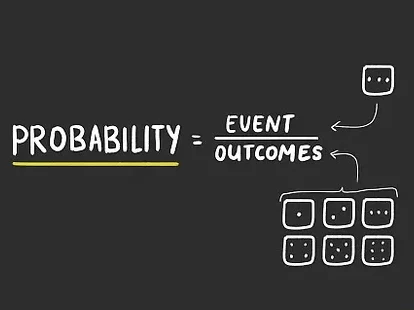Understanding probability rules is essential for solving probability problems. There are a number of rules applicable: multiplication rule, addition rule, law of the compliment and conditional probabilities. We also call the rules ‘basic relationships of probability’.

We use this rule when we want to know the possibility of occurrence of at least one of two or more events. That is, with events A and B, we want to know the probability of A or B or even the two events occurring.

Recall the types of events we discussed yesterday, this come into play. The addition rule deals with ‘mutually exclusive events’ and ‘non mutually exclusive events’.

For non-mutually exclusive events, the addition rule is the probability of event A occurring plus the probability of B occurring minus commonalities between the two events.

This is denoted as P(AuB) = P(A) + P(B)- P(AnB) for not mutually exclusive events and P(AuB) = P(A) + P(B) for mutually exclusive events.

For additional probability rule; ‘or’ ‘either’ are commonly used to know this rule is applicable. where it is not expressly stated, it is implied.

## The multiplication rule

The multiplication rule is used to find the probability of two events occurring in sequence.

Probability questions for multiplication rule often use key words such as ‘and’, ‘both’. For instance, what is the probability that event A and event B will occur? better still, what is the probability of both A and B occurring?

Multiplication rule is for independent and conditional probabilities. For independent events, multiplication rule is denoted as P(A) x P(B) but for conditional events it is denoted as P(A) x P(B|A).

## Law of the compliment

The compliment of an event A is the event(s) of all the sample points in the sample space that are not in event A. That is, all events in the sample aside A, are compliments of A.

The sum of event A and all it’s compliments equals 1. Therefore, we can denote the rule as P(A) = 1 – P(A’) where A’ is compliment of A.

## Conditional Probability

We use this to determine the probability of an event, given that another event has occurred. That is, with the knowledge of occurrence of a probability, we can know if the other has the likelihood of occurrence.

It is usually denoted as P(A|B) – which can be read as probability of A given B. And P(A|B) = P(AnB) divided by P(B). From the formula, we want to know the probability of A because we already know the probability of B.

Let us consider an example. In an organization with 960 male workers and and 240 female workers; the management promote 288 male and 36 female workers. We want to find the probability of a worker getting promotion as a result of being:

a) a male worker

b) a female worker

First, we need to know the probability of male P(M), probability of female P(F), then we get the intersection and then the conditional probabilities.

P(M) is total male divide by total workers; that is, 960 divided by 1,200 which equals 0.8. This means, if you pick a worker at random, the chance of picking a male is 80%.

P(F) is total female divide by total workers, that is, 240 divided by 1,200 which equals 0.2. This means, if you pick a worker at random, the chance of picking a female is 20%.

To be continue…## AWOL & Ajaokuta Steel Mill – April 21 to 23.

in